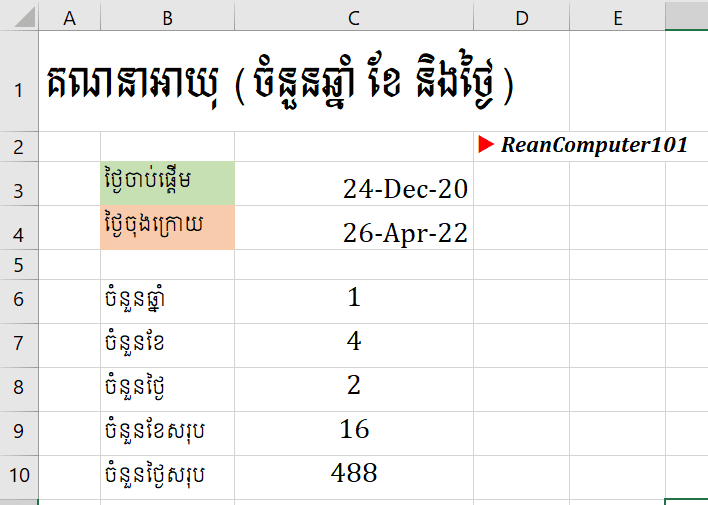# [New] How to Calculate Age in Excel with Number of Years Months and Days

### How to Calculate Age in Excel with Number of Years Months and Days

If you have two different date in Microsoft Excel and you want to calculate as the following:
- Total Days between two dates
- Total Months between two dates
- Find Age between two date like number of Year with month and daysThis Excel tutorial in khmer help you do that in the video using DateDif formula step by step and you can download Excel example file down below.
DateDif formula in Excel
=DATEDIF (start_date, end_date, format)
◾ start_date: Required, start date you want to calculate.
◾ end_date: Required, end date you want to calculate.
◾ Format: required
- "y" Difference in complete years
- "m" Difference in complete months
- "d" Difference in days
- "md" Difference in days, ignoring months and years
- "ym" Difference in months, ignoring years
- "yd" Difference in days, ignoring years

WATCH MORE HOW TO FORMAT DATE IN KHMER IN EXCEL